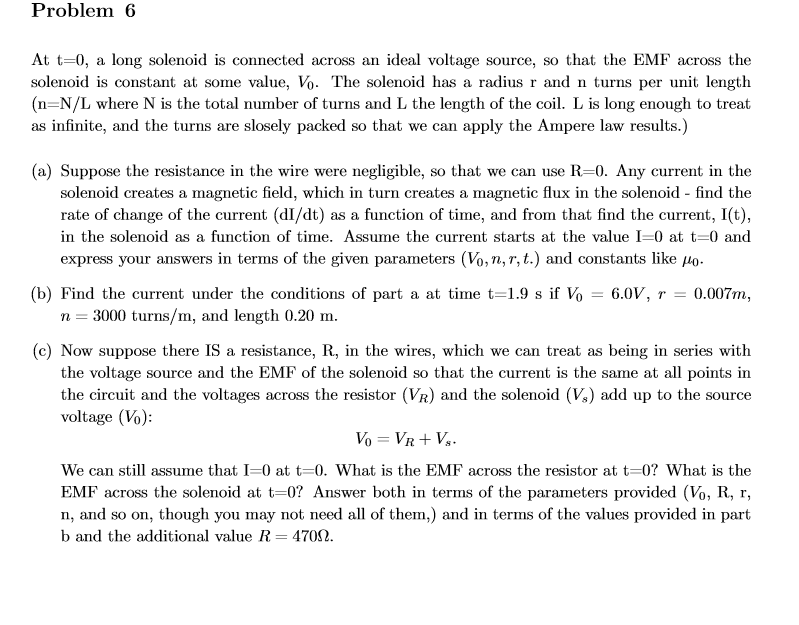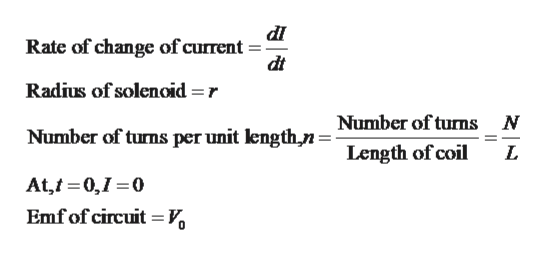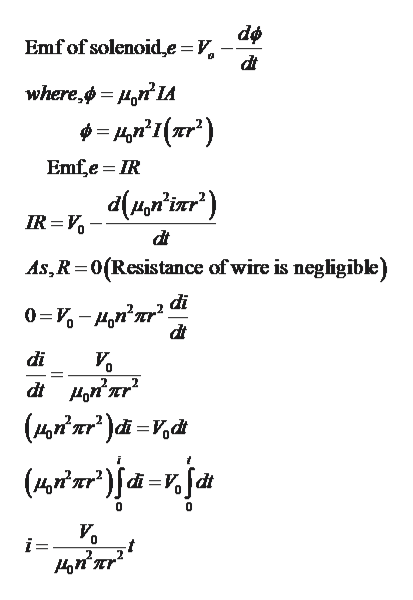# Problem 6At t-0, a long solenoid is connected across an ideal voltage source, so that the EMF across thesolenoid is constant at some value, Vo. The solenoid has a radius r and n turns per unit length(n-N/L where N is the total number of turns and L the length of the coil. L is long enough to treatas infinite, and the turns are slosely packed so that we can apply the Ampere law results.)(a) Suppose the resistance in the wire were negligible, so that we can use R-0. Any current in thesolenoid creates a magnetic field, which in turn creates a magnetic flux in the solenoid - find therate of change of the current (dI/dt) as a function of time, and from that find the current, I(t),in the solenoid as a function of time. Assume the current starts at the value I=0 at t-0 andexpress your answers in terms of the given parameters (Vo,n,r, t.) and constants like o.0.007m(b) Find the current under the conditions of part a at time t=1.9 s if Vo = 6.0V, r =3000 turns/m, and length 0.20 m.(c) Now suppose there IS a resistance, R, in the wires, which we can treat as being in series withthe voltage source and the EMF of the solenoid so that the current is the same at all points inthe circuit and the voltages across the resistor (VR) and the solenoid (Vs) add up to the sourcevoltage (Vo)SVo VRWe can still assume that I=0 at t-0. What is the EMF across the resistor at t=0? What is theEMF across the solenoid at t-0? Answer both in terms of the parameters provided (Vo, R, r,n, and so on, though you may not need all of them,) and in terms of the values provided in partb and the additional value R= 4700

Question
4 viewshelp_outlineImage TranscriptioncloseProblem 6 At t-0, a long solenoid is connected across an ideal voltage source, so that the EMF across the solenoid is constant at some value, Vo. The solenoid has a radius r and n turns per unit length (n-N/L where N is the total number of turns and L the length of the coil. L is long enough to treat as infinite, and the turns are slosely packed so that we can apply the Ampere law results.) (a) Suppose the resistance in the wire were negligible, so that we can use R-0. Any current in the solenoid creates a magnetic field, which in turn creates a magnetic flux in the solenoid - find the rate of change of the current (dI/dt) as a function of time, and from that find the current, I(t), in the solenoid as a function of time. Assume the current starts at the value I=0 at t-0 and express your answers in terms of the given parameters (Vo,n,r, t.) and constants like o. 0.007m (b) Find the current under the conditions of part a at time t=1.9 s if Vo = 6.0V, r = 3000 turns/m, and length 0.20 m. (c) Now suppose there IS a resistance, R, in the wires, which we can treat as being in series with the voltage source and the EMF of the solenoid so that the current is the same at all points in the circuit and the voltages across the resistor (VR) and the solenoid (Vs) add up to the source voltage (Vo) S Vo VR We can still assume that I=0 at t-0. What is the EMF across the resistor at t=0? What is the EMF across the solenoid at t-0? Answer both in terms of the parameters provided (Vo, R, r, n, and so on, though you may not need all of them,) and in terms of the values provided in part b and the additional value R= 4700 fullscreen
check_circle

Step 1

Given,help_outlineImage TranscriptionclosedI Rate of change of current- dt Radius of solenoid =r Number of turns N Number of turns per unit lengthn- Length of coil L At,t 0,1 0 Emfof circuit V fullscreen
Step 2

Expression of current in terms of t can be derived as follows,help_outlineImage TranscriptioncloseEmfof solenoide = V where,ф - pт'и Emfe IR IR V As,R 0(Resistance of wire is negligible) di 03D - нап'ar? di 2 dt (wiwrija-nja 2 2 тar fullscreen
Step 3

Part (b):

Give...

### Want to see the full answer?

See Solution

#### Want to see this answer and more?

Solutions are written by subject experts who are available 24/7. Questions are typically answered within 1 hour.*

See Solution
*Response times may vary by subject and question.
Tagged in

### Physics